# VALIDATION OF NATURAL CONVECTION

The famous experiment of Nukiyama, carried out in 1934, was the first to classify the different regimes of pool boiling as shown in the figure below (Global Digital Central Encyclopedia, Thermal fluid central ). He observed that the bubble did not appear until the $\Delta T \approx 5°C$ in which $T_{wall} = \Delta T + T_{sat}$ and this particular wall temperature is referred as $T_{ONB}$ (the onset nucleate boiling, ONB). For $T_{wall}<T_{ONB}$, free convection boiling is expected to be the only mechanism for the system to evacuate heat to the surrounding domain.Figure 10: Pool boiling curve for saturated water, according to Global Digital Central Encyclopedia, Thermal fluid central 

The boiling curve for propane, in logarithmic scale, is presented in Figure 3 (BIBLIOGRAPHIC RESEARCH - Boiling Regime section). The free convection boiling is said to exist for $\Delta T < 7°C$, thus for heat fluxes lower than 2000W/m² before a region of transition regime that ranges between 2000 - 5000 W/m².

Therefore, single phase simulations were launched for various heat flux, from 200 to 5000 W/m². As discussed in the 'BIBLIOGRAPHIC RESEARCH - Boussinesq Approximation' section, the flow generated around the tube due to buoyancy force, as a result of variation of propane density, was estimated using Boussinesq Approximation. Results obtained from Neptune CFD were compared with correlations and experimental results in the 'Comparison' section to conclude on the reliability of Boussinesq Approximation and Neptune CFD to predict the fluid movement around the tube for natural convection boiling regime.

# Correlation

The studied domain was limited to a single isolated cylinder tube in a pool of static propane as demonstrated in 'MESH' section. The $\Delta T$ is less than 6K for natural convection regime, hence the variation of density remains small and can be calculated with Boussinesq approximation (Equation 2). Considering the value of Rayleigh number for this maximum $\Delta T$, calculated in 'BIBLIOGRAPHIC RESEARCH Boussinesq approximation' section, laminar regime is adopted.

In the literature, the Correlations by Morgan (1975)  and by Churchill and Chu (1975)  are found to be well adapted for the range of Rayleigh number $Ra_D$ obtained for $\Delta T$ lying between 1 - 6K.

Correlation by Morgan(1975) :

$Nu_D= \frac{hD}{k}=CRa_D^n$   (37)

in which C=0.125, n=0.333, for $Ra_D~10⁷ - 10^{12}$

Correlation by Churchill and Chu (1975) :

$Nu_D=(0.60+\frac{0.387Ra_D^{\frac{1}{6}}}{(1+(\frac{0.559}{Pr})^{\frac{9}{16}})^{\frac{8}{27}}})^2$   (38)

for $Ra_D<10^{12}$

And considering also the classical equation of heat transfer

$Q_{wall}=h\Delta T$   (39)

# Neptune CFD : Monophasic simulation

In this phase of study, simulations were carried out for the range of temperature difference mentioned before by imposing a heat flux desity as boundary condition for the heating tube. The simulations were launched in saturation condition with Psat=474.3 kPa. The Boussinesq hypothesis (Equation 2 of BIBLIOGRAPHY RESEARCH - Boussinesq Approximation) was used to predict the variation of density due to ΔT, thus the flow in the studied domain caused by the difference of buoyancy force.

In order to set the properties and conditions, the interface of Neptune CFD, called EDAMOX, was used. It was developed for users to define and select different models as well as boundary conditions to be used during their simulation. The interface can be accessed by the following command in linux terminal:

edam &

or if the param file already exists

edam –U param &

Note that one has to be in ‘DATA’ directory to launch Edamox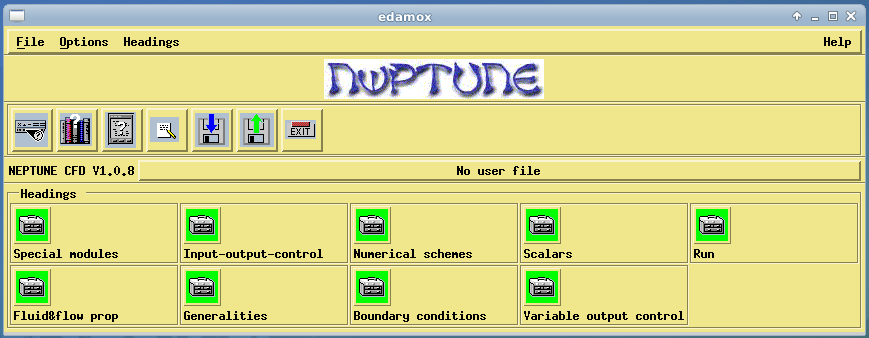Figure 11: Edamox interface

The first step of the simulation definition was to select the special module in case of necessity. For single phasic simulation, no specific module has to be selected.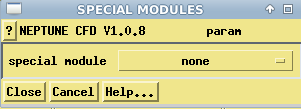Figure 12: Neptune Special module panel for natural convection

In Fluid & Flow prop windows, the properties of the fluid at the reference temperature are required. In this study, they were fixed at Tsat at Psat=474.3kPa. Since the propone was at static state, no turbulence was expected in the simulation. (see also Rayleigh Calculation in BIBLIOGRAPHIC RESEARCH, Boussinesq approximation)

The mesh file needs to be defined in input-output-control windows. The number of iteration and time of the simulation are required, as well as the output frequency, for post-processing.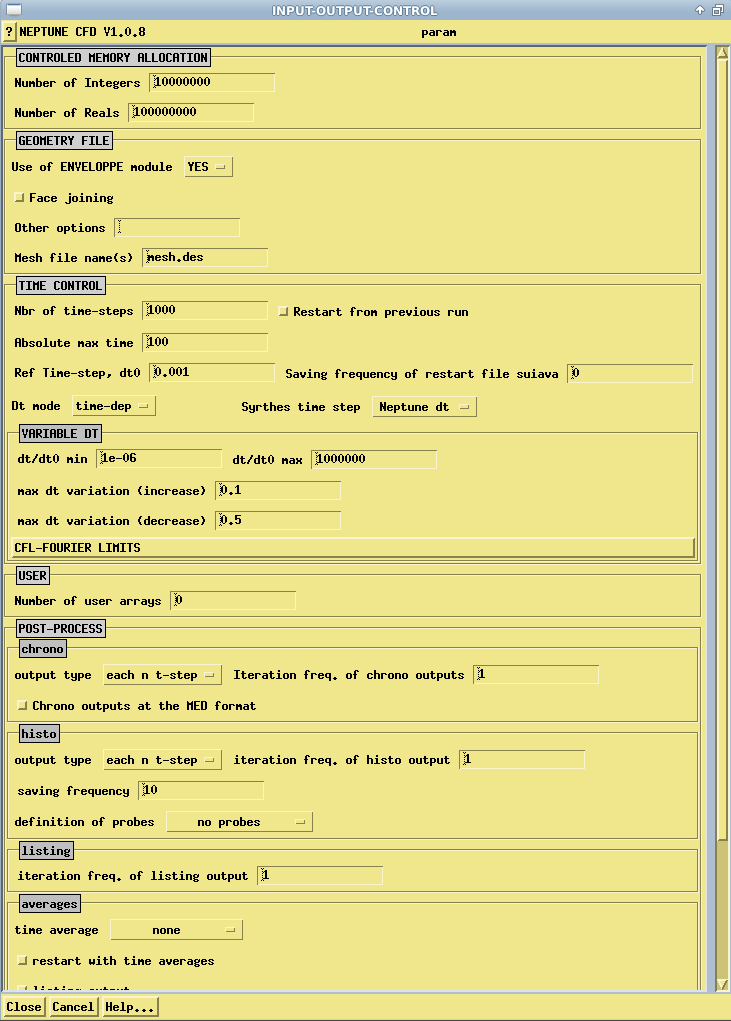Figure 13: Neptune Input-Output-Control panel for natural convection

In order to take into account the variation of density, modifications in USPHYV.F are required to impose the Boussinesq Approximation and, consequently, the option ‘Variable physical properties (call USPHYV)’ has to be selected in the Physical models module of param file.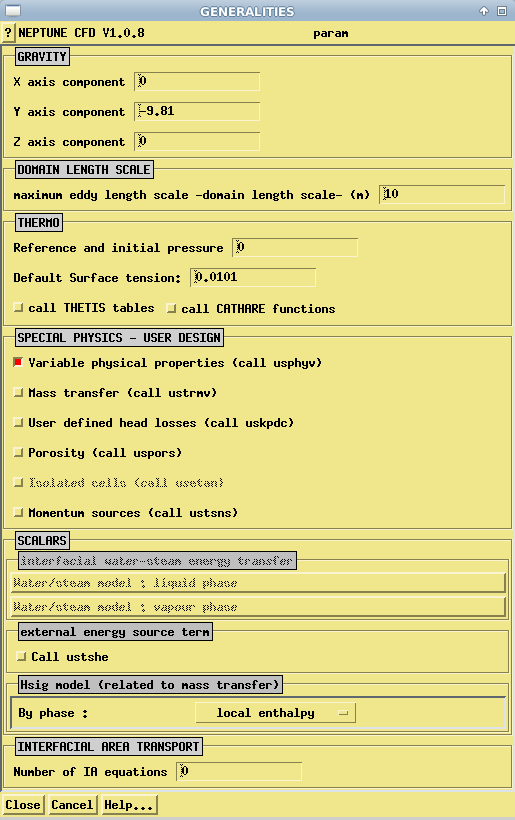Figure 14: Neptune Generalities panel for natural convection

In the Scalars windows, a total enthalpy scalar type was selected in order to be able to fix either a constant heat flux [W/m²] or impose a wall temperature as boundary condition around the tube. The parameter ‘lam.dym.coef’, corresponds in this case to the thermal conductivity of propane [W/m/K] over the specific heat capacity of propane [J/kg/K], as the scalar type is chosen to be total enthalpy.

However, the wall temperature, Tw and wall heat flux, Qwall cannot be accessed if the water/steam special module is not selected, as Neptune CFD does not calculate them for single phasic simulation. Therefore, a wall temperature was imposed, at the first place, as the boundary condition around the tube and the mesh was refined until the temperature of the first mesh around the tube was approximately (with a difference of 0.001) equal to the applied wall temperature. The wall heat flux could be easily accessed from the ‘listing’ file by dividing the energy transfer around the tube by the exchange surface. To impose a wall temperature, a dirichlet boundary condition has to be selected and the value imposed has to be in the same unit as the scalar. One should never considered the 'Timp[K]' option for boundary condition if either the cathare or thesis tables are not selected.

The refined mesh was used for simulations with various heat fluxes imposed, range from 300 W/m2 to 5000 W/m2, as the wall boundary condition. Using ‘Paraview’, a data analysis and visualisation application, the liquid temperature of the first mesh can be exported using the ‘slices’ function (Figure 15). Average wall temperature can, hence, be obtained by an integration of the exported data.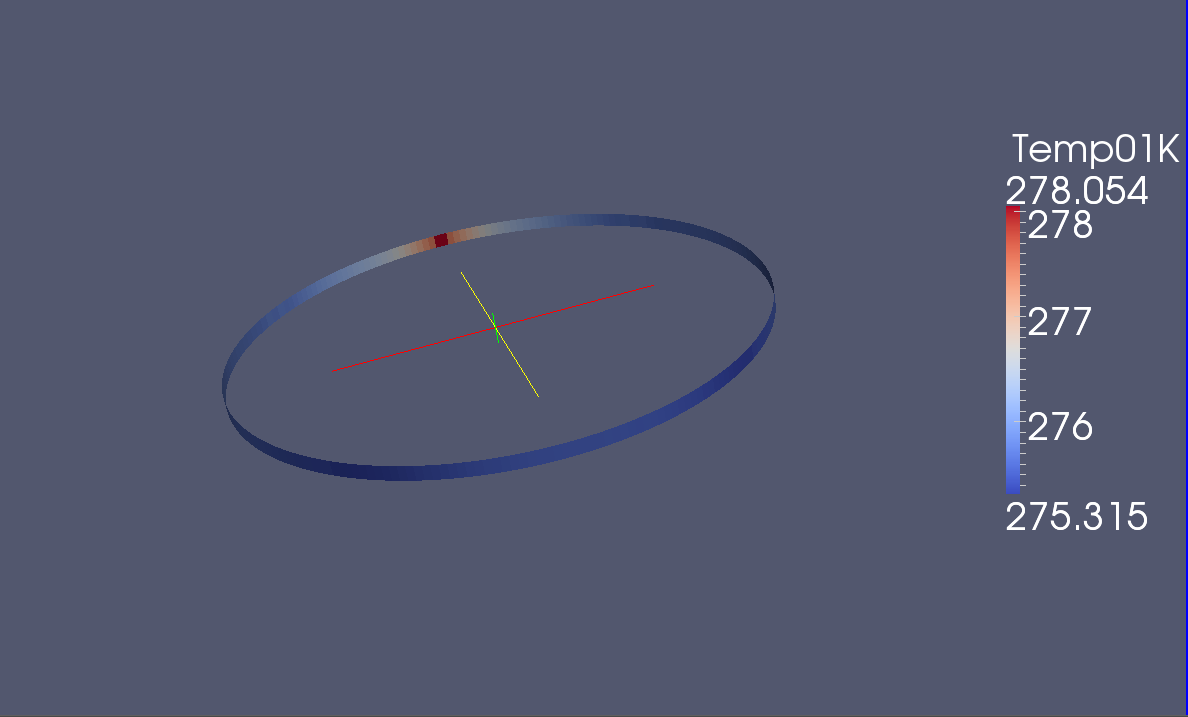Figure 15: Slice of the geometry, Twall calculation for natural convection

# Comparison

The comparison between the simulation results, results predicted by correlations and experimental results is plotted below:Figure 16: Comparison between simulation results, experimental results  and correlations  for natural convection

As shown in the figure above (Figure 16) the results predicted by Neptune_CFD are very close to the experimental results, where the difference is estimated to be lower than 15% for a heat flux up to 2000 W/m2. Further from 2000 W/m2, the simulation results and even those obtained by the correlations are no longer comparable with experimental results. By referring to the boiling curve of propane (Figure 3 in ‘BIBLIOGRAPHY RESEARCH’ section), it is said that a transition regime exists between 2000 - 5000 W/m2 before the boiling takes place. Therefore, the incoherence between results is expected as the simulations and correlations are initially developed for natural convection regime.

Consequently, the simulation results by Neptune_CFD are proved to be reliable and the Boussinesq hypothesis is well-adapted to predict the natural convection for propane while for higher heat flux, the nucleate boiling model has to be taken into consideration.